• 简单概括，流程型与离散型有如下不同？ 流程型制造使用配方或食谱。 离散制造使用物料清单（BOM）。 离散制造商顺着工艺路线进行组装，而流程制造商则多批量混合。 因此，离散制造商 - 包括按库存生产，按订单生产...简单概括，流程型与离散型有如下不同？

流程型制造使用配方或食谱。 离散制造使用物料清单（BOM）。 离散制造商顺着工艺路线进行组装，而流程制造商则多批量混合。 因此，离散制造商 - 包括按库存生产，按订单生产和组装订生产需要复杂周全的计划，调度和跟踪功能，以改善运营和盈利能力。

而具有批量或连续操作的流程型制造商依赖于复杂的跟踪和调配机制来保证高效的加工执行。

离散制造就是组装东西，制作精确的东西。 这些产品通常以单独定义的批次生产，工作中心的顺序跟随生产而变化。 因此，在离散制造中，产品通过使用相同的工艺路线或由相同操作者按顺序逐步加工制成。 基于生产订单和产品的离散制造经常从一个订单到另一个订单。

而流程制造是指您的产品需要完成一组流程，而每个流程都需要某些要求，因此，在规划和制定制造要求时，最好将每个流程与另一个流程分开。如果单独处理，则进行控制和维护。流程型制造的东西不能进行分拆，从技术上讲，你可以将混合物从容器中取出并重新使用容器，但你不能从生产的成品中取出原料。这使得流程制造的过程基于配方。离散型制造商正在生产可能有螺钉，螺母，手柄等的成品，如果需要可以将其拆开并用于其他用途。在离散制造中，生产车间按照车间订单（计划）来制造某些东西。个别产品易于识别。在流程制造过程中，您无法分辨出一种产品与另一种产品之间的区别。

这两个不同制造领域之间的最大区别在于，离散制造的产物可以毫无困难地逆转，而对于流程制造而言，没有办法做到这一点。流程制造涉及可变成分，副产品和副产品，而离散制造则是标准零件和部件驱动。离散制造使用复杂多级BOM，而流程制造使用多个配方和公式。流程制造的必要条件是Lot Potency和Shelf Life，而离散制造价值序列号，ECN和组装。流程型制造的特征在于生产方法，其在任何一个生产过程中或在类似产品的生产过程的实际处理中具有最小化的中断。但是，离散制造的特点是生产不同的物品，这些物品使用物料清单和工艺路线来确定成本和交货时间。

大多数加工业的特点是制造大量产品的行业，例如油漆，药品，饮料和食品，这些产品经常进行化学转化。另一方面，离散制造商生产或组装可识别为不同单元的组件或成品，例如汽车，能够被序列化或标签产品识别并且可评估为数量而不是重量或体积。

在流程工业中，我们没有学会做面向缺陷的制造，比如说如果你加工过多的染料，你可以放一些基础并修复它的方法

流程型是直接的，实时的控制。而在离散制造中，其事后统计分析可以得到持续改进。


展开全文Process 流程
• ML之LF：机器学习、深度学习中常见的损失函数(Loss... 损失函数，又称目标函数，或误差函数，用来度量网络实际输出期望输出之间的不一致程度，指导网络的参数学习和表示学习。 0、损失函数特点 损失函数是一个非...
ML之LF：机器学习中常见的损失函数(连续型/离散型)的简介、损失函数/代价函数/目标函数之间区别、案例应用之详细攻略

目录

损失函数的简介

损失函数/代价函数/目标函数之间区别

损失函数的案例应用

损失函数的简介

损失函数，又称目标函数，或误差函数，用来度量网络实际输出与期望输出之间的不一致程度，指导网络的参数学习和表示学习。

0、损失函数特点

损失函数是一个非负实值函数。
针对不同的问题，会采用不同的损失函数
– 回归问题(连续型)：平方损失等
– 分类问题(离散型)：对数损失、交叉熵等
不同的损失函数会影响网络的训练速度和网络的泛化性能
1、损失函数-连续型输出

平方损失函数(Square Loss)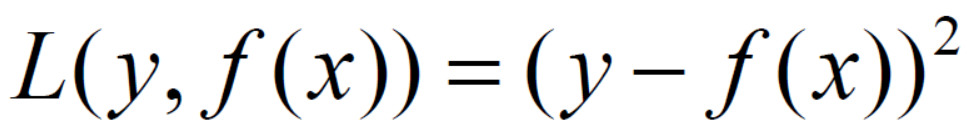绝对值损失函数(Absolute Value Loss)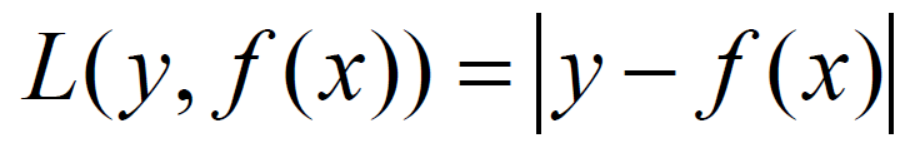2、损失函数-离散型输出

交叉熵损失(Cross-Entropy Loss； Log Loss)，交叉熵损失：真实概率?? ; 模型预测概率??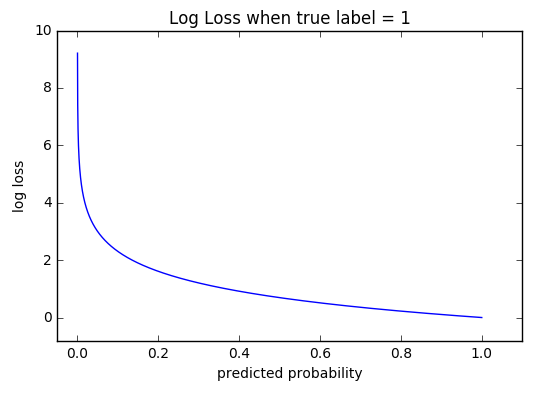熵：用于度量变量的不确定性程度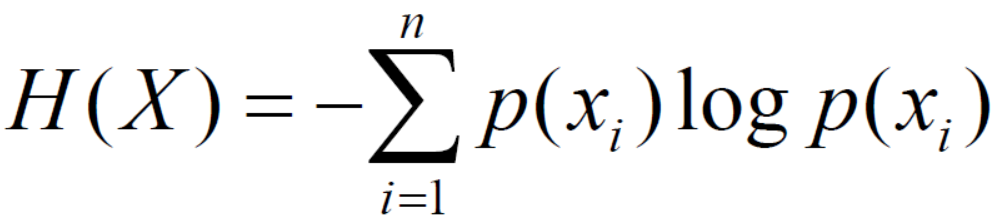交叉熵：主要用于度量两个概率分布间的差异性信息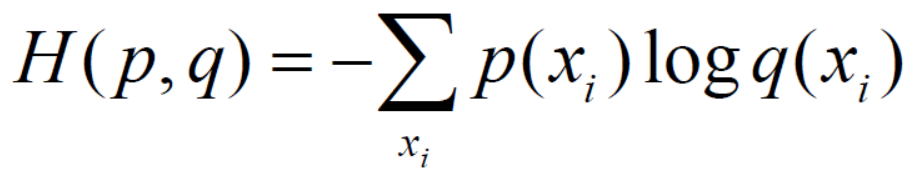(1)、二分类问题的交叉熵损失函数：
对于样本（?, ?），?为样本， ?为对应的标签, 在二分类问题中，其取值的集合可能为{0，1}。假设某个样本的真实标签为?，该样本的? = 1的概率为?，则该样本的损失函数为：− (????(?) + 1 − ? log(1 − ?))。

2.1、交叉熵和Softmax在多分类问题的结合应用

ML之SR：Softmax回归(Softmax Regression)的简介、使用方法、案例应用之详细攻略

损失函数/代价函数/目标函数之间区别

损失函数

用来估计你模型的预测值与真实值Y的不一致程度，它是一个非负实数值函数。损失函数越小，模型的鲁棒性就越好。是定义在单个样本上的，算的是一个样本的误差。

(1)、均方误差：也叫平方损失，是回归任务中最常见的性能度量。我们把均方误差当做线性回归的损失函数。因此我们可试图让其最小化

代价函数
定义在整个训练集上的，是所有样本误差的平均，也就是损失函数的平均。
目标函数

定义为最终需要优化的函数。等于结构风险Cost Function+正则化项。正则化项：我们直接最小化经验风险很容易产生过拟合现象，所以一般需要在经验风险上加正则化项或损失，结构风险定义为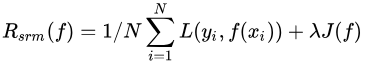其中为模型的复杂度。模型越复杂，复杂度越大。复杂度表示了对复杂模型的惩罚。结构风险最小化，需要经验风险与模型复杂度同时最小。

损失函数的案例应用

后期更新……


展开全文• 离散型连续型特征列的选择 一、CART分类树（待预测结果为离散数据）： 选择具有最小Gain_GINI的属性及其属性值，作为最优分裂属性以及最优分裂属性值。Gain_GINI值越小，说明二分之后的子样本的“纯净度”越高...
CART分类回归树
分类与回归树是二叉树，可以用于分类，也可以用于回归问题。
区别：分类树输出的是样本的类别， 而回归树输出的是一个实数。
对离散型和连续型特征列的选择
一、CART分类树（待预测结果为离散数据）：
选择具有最小Gain_GINI的属性及其属性值，作为最优分裂属性以及最优分裂属性值。Gain_GINI值越小，说明二分之后的子样本的“纯净度”越高，即说明选择该属性（值）作为分裂属性（值）的效果越好。
实现过程：

对于样本集S，计算GINI如下：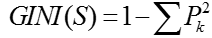其中，在样本集S中，pk表示分类结果中第k个类别出现的频率；
对于含有N个样本的样本集S，根据属性A的第i个属性值，将数据集S划分成两部分，则划分成两部分之后，Gain_GINI计算如下：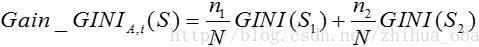对于属性A，分别计算任意属性值将数据集划分成两部分之后的Gain_GINI，选取其中的最小值，作为属性A得到的最优二分方案；
对于样本集S，计算所有属性的最优二分方案，选取其中的最小值，作为样本集S的最优二分方案：所得到的属性A及其第i属性值，即为样本集S的最优分裂属性以及最优分裂属性值。

举个简单的例子，如下图：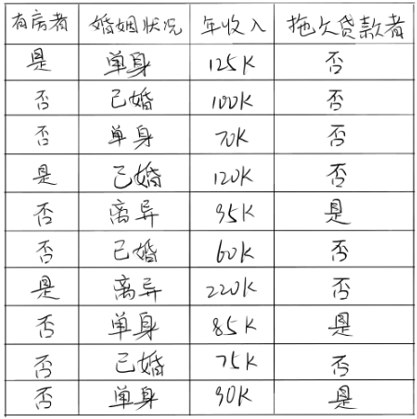在上述图中，属性有3个，分别是有房情况，婚姻状况和年收入，其中有房情况和婚姻状况是离散的取值，而年收入是连续的取值。拖欠贷款者属于分类的结果。
假设现在来看有房情况这个属性，那么按照它划分后的基尼增益计算如下：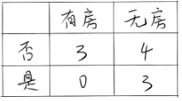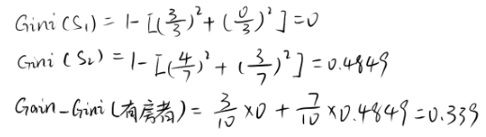而对于婚姻状况属性来说，它的取值有3种，按照每种属性值分裂后基尼增益计算如下: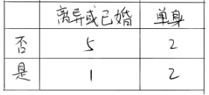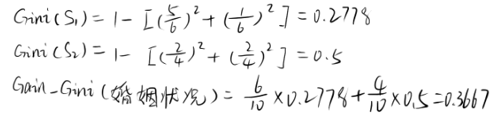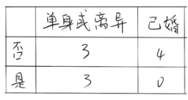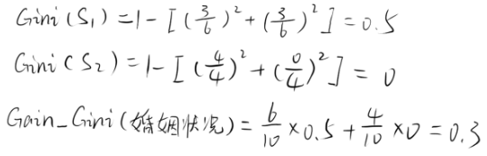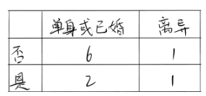比较上述三种取值得到的基尼增益可知，以“已婚”这个取值来分裂婚姻状况属性是最好的。
二、CART回归树（待预测结果为连续型数据）
区别于分类树选取Gain_GINI为评价分裂属性的指标，回归树选取Gain_σ（方差）为评价分裂属性的指标。选择具有最小Gain_σ的属性及其属性值，作为最优分裂属性以及最优分裂属性值。Gain_σ值越小，说明二分之后的子样本的“差异性”越小，说明选择该属性（值）作为分裂属性（值）的效果越好。
实现过程：

对于含有连续预测结果的样本集S，总方差计算如下：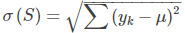其中，μ表示样本集S中预测结果的均值，yk表示第k个样本预测结果；
对于含有N个样本的样本集S，根据属性A的第i个属性值，将数据集S划分成两部分，则划分成两部分之后，Gain_σ计算如下：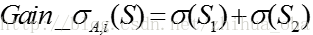对于属性A，分别计算任意属性值将数据集划分成两部分之后的Gain_σ，选取其中的最小值，作为属性A得到的最优二分方案；
对于样本集S，计算所有属性的最优二分方案，选取其中的最小值，作为样本集S的最优二分方案：所得到的属性A及其第i属性值，即为样本集S的最优分裂属性以及最优分裂属性值。

继续拿上面那个数据集来讲解上述的步骤：
1、	根据第1个属性值划分为<=125和>125两部分：
<=125的有：125, 100, 70, 120, 95, 60, 85, 75, 90    均值为91.111
>125的有：220    均值为220
σ(S1) = 61.554
σ(S2) = 0
Gain_σ(S) = σ(S1) + σ(S2) = 61.554
2、	根据第2个属性值划分为<=100和>100两部分：
<=100的有：100, 70, 95, 60, 85, 75, 90    均值为82.143
而>100的有：125,120,220    均值为155
σ(S1) = 35.254
σ(S2) = 79.687
Gain_σ(S) = σ(S1) + σ(S2) = 114.941
3、	根据第3个属性值划分为<=70和>70两部分：
Gain_σ(S) = σ(S1) + σ(S2) =129.085
4、	根据第4个属性值划分为<=120和>120两部分：
Gain_σ(S) = σ(S1) + σ(S2) =117.144
5、	根据第5个属性值划分为<=95和>95两部分：
Gain_σ(S) = σ(S1) + σ(S2) =122.347
6、	根据第6个属性值划分为<=60和>60两部分：
Gain_σ(S) = σ(S1) + σ(S2) =128.798
7、	根据第7个属性值划分为<=220和>220两部分：
Gain_σ(S) = σ(S1) + σ(S2) =136.894
8、	根据第8个属性值划分为<=85和>85两部分：
Gain_σ(S) = σ(S1) + σ(S2) =126.656
9、	根据第9个属性值划分为<=75和>75两部分：
Gain_σ(S) = σ(S1) + σ(S2) =125.568
10、	根据第10个属性值划分为<=90和>90两部分：
Gain_σ(S) = σ(S1) + σ(S2) =125.511
比较每一种划分得到的Gain_σ(S)可知，用“125”来划分“年收入”这个属性是最合适的。
这样一来，连续型的这个属性也可以被划分为两部分：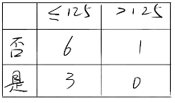计算它的基尼增益：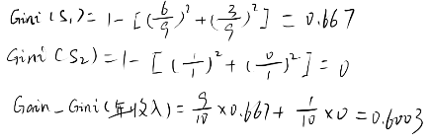到此每个属性的最优二分方案均已计算出，比较每个属性的基尼增益可得出：此数据集的最优分裂属性为“婚姻状况”（因为这个属性的基尼增益值最小，为0.3），以及最优分裂属性值为“已婚”。


展开全文• 离散型随机变量和连续型随机变量最大的区别在于二者在概率计算上是不同的 对于离散型随机变量，概率函数f(x)f(x)f(x)给出了随机变量xxx取某个特定值的概率 而对于连续型随机变量 概率函数相对应的是概率密度 也记...
连续型概率分布

离散型随机变量和连续型随机变量最大的区别在于二者在概率计算上是不同的 对于离散型随机变量，概率函数f(x)$f\left(x\right)$$f(x)$给出了随机变量x$x$$x$取某个特定值的概率 而对于连续型随机变量 与概率函数相对应的是概率密度 也记作f(x)$f\left(x\right)$$f(x)$

连续型随机变量，通过给定区间上曲线f(x)$f\left(x\right)$$f(x)$下的面积给出连续型随机变量在该区间取值的概率

连续型概率分布主要有:均匀概率分布 正态分布 指数分布

均匀概率分布

定义：一种连续型概率分布，随机变量在等长度的区间上取值的概率相同
均匀概率密度函数：
f(x)=1b−a,a≤x≤b$f\left(x\right)=\frac{1}{b-a},a\le x\le b$$f(x) = \frac {1}{b-a},a \leq x \leq b$  或 f(x)=0,其他$f\left(x\right)=0,其他$$f(x) = 0 ,其他$
连续型均匀概率分布：
数学期望为： E(x)=(a+b)2$E\left(x\right)=\frac{\left(a+b\right)}{2}$$E(x) = \frac {(a+b)}{2}$
方差为： Var(x)=(b−a)212$Var\left(x\right)=\frac{\left(b-a{\right)}^{2}}{12}$$Var(x) = \frac {(b-a)^2}{12}$

正态概率分布

正态概率分布：是描述连续型随机变量的最重要的一种概率分布 其概率密度函数呈钟形 由均值u$u$$u$和标准差σ$\sigma$$\sigma$确定

正态概率密度函数：
f(x)=12π√σe−(x−u)2/2σ2$f\left(x\right)=\frac{1}{\sqrt{2\pi }\sigma }{e}^{-\left(x-u{\right)}^{2}/2{\sigma }^{2}}$$f(x) = \frac {1}{\sqrt{2\pi}\sigma} e^{-(x-u)^2/2\sigma^2}$
其中：u$u$$u$为均值，σ$\sigma$$\sigma$为标准差 π$\pi$$\pi$为3.14159 e$e$$e$为2.71828

正态分布特征

（1） 正态分布族中的每个分布因均值u$u$$u$和标准差σ$\sigma$$\sigma$这两个参数的不同而不同
（2） 正态曲线的最高点在均值处达到，均值还是分布的中位数和众数
（3） 分布的均值可以是任意数据：负数、零或正数
（4） 正态分布永远是对称的
（5） 标准差决定曲线的宽度和平坦程度
（6） 正态随机变量的概率由正态曲线下的面积给出
（7） 下面是随机变量在一些常用区间内取值的百分比
a. 正态随机变量有68.3%的值在均值加减一个标准差的范围内
b. 正态随机变量有95.4%的值在均值加减两个标准差的范围内
c. 正态随机变量有99.7%的值在均值加减三个标准差的范围内

标准正态概率分布

定义：随机变量服从均值0并且标准差为1的正态分布 则称该随机变量服从标准正态概率分布
标准正态密度函数： f(z)=12π√e−z2/2$f\left(z\right)=\frac{1}{\sqrt{2\pi }}{e}^{-{z}^{2}/2}$$f(z) = \frac {1}{\sqrt{2\pi}} e^{-z^2/2}$

正态分布数学用表P(z≤1.0)=0.8413$P\left(z\le 1.0\right)=0.8413$$P(z\leq1.0) = 0.8413$
计算三种类型的概率：（1）标准正态随机变量z$z$$z$小于或等于某个给定值的概率 （2）z$z$$z$在两个给定值之间的概率 （3）z$z$$z$大于或等于某个给定值的概率

随机正态概率分布的概率：

转化为标准正态随机变量： z=(x−u)σ$z=\frac{\left(x-u\right)}{\sigma }$$z = \frac {(x-u)}{\sigma}$

二项概率的近似分布

连续性修正因子：当用连续性正态概率分布来近似离散型二项概率分布时 从xx中加上或减去的值0.5
例如：发票出错10% 100次中 恰有12次出错的概率 P(11.5≤x≤12.5)$P\left(11.5\le x\le 12.5\right)$$P(11.5 \leq x \leq 12.5)$

指数概率分布

作用：用于描述诸如到达某洗车处的两辆车的时间间隔 装载一辆卡车所需时间、告诉公路上两起重大事故发生地之间的距离等随机变量

指数概率密度函数： f(x)=1ue−x/u,x≥0$f\left(x\right)=\frac{1}{u}{e}^{-x/u},x\ge 0$$f(x) = \frac {1}{u}e^{-x/u}, x\geq 0$
u$u$$u$为期望值

指数分布：累积概率
P(x≥x0)=1−e−xo/u$P\left(x\ge {x}_{0}\right)=1-{e}^{-{x}_{o}/u}$$P(x\geq x_0) = 1 - e^{-x_o/u}$

泊松分布与指数分布的关系

泊松分布：描述了每一区间中事件发生的次数
指数分布：描述了事件发生的时间间隔长度
展开全文统计学
• 企業的生產方式,主要可以分為按定單生產,按庫存生產或上述兩者的組合. 從生產類上考慮,則可以分為批量生產和單件小批生產. ...既有批量生產,也有單件小批生產.（流程制造包括重复生产和连续生产）
• 一、CARTID3的区别 通过之前的研究发现，CARTID3算法...ID3和CART算法的区别主要集中在树的生成和树的修剪方面，但是ID3算法只能处理离散型的描述性属性。C4．5算法是ID3算法的后续算法，它能够处理连续型数据。算法
• 前馈神经网络取连续离散变量，一般不考虑输出输入在时间上的滞后效应，只表达输出输入的映射关系； 反馈神经网络可以用离散变量也可以用连续取值，考虑输出输入之间在时间上的延迟，需要用动态方程来...
• 离散型随机变量和连续型随机变量之间最根本的区别在于，二者在概率计算上是不同的。对一个离散型随机变量，概率函数f（x）给出了随机变量x取某个特定值的概率。而对连续型随机变量，概率函数相对应的是概率密度...统计学 数据分析
• 分类回归的区别在于输出的类型： 分类：分类问题输出的是离散型变量，比如（-1，+1），输出一种类别，可看做定性输出 回归：输出的是连续型变量，可看作定量输出 回归优化的对象是让平方误差最小 分类回归的对象是...
• 离散型变量和连续型变量2. 概率分布概率函数3. 概率分布函数概率函数函数4. 连续型变量的概率、概率分布函数、概率密度函数之间的关系 声明 本文主要内容转载至博主马尔代夫Maldives的简书概率函数P(x)、概率...
• PDF：概率密度函数（probability density function）, 在数学中，连续型随机变量的概率密度函数（在不至于混淆时可以简称为密度函数）是一个描述这个随机变量的输出值，在某个确定的取值点附近的可能性的函数。...
• 在模型训练过程中，我们会对训练数据集进行抽象、抽取大量特征，这些特征中有离散型特征也有连续型特征。若此时你使用的模型是简单模型（如LR），那么通常我们会对连续型特征进行离散化操作，然后再对离散的特征，...
• 参考博客：离散型特征编码方式：one-hot哑变量* 一、导语 在机器学习问题中，我们通过训练数据集学习得到的其实就是一组模型的参数，然后通过学习得到的参数确定模型的表示，最后用这个模型再去进行我们后续的...机器学习 python
• 连续型时间数据：事实上连续型时间数据和离散型时间数据没有本质区别，因为时间是可以不断细分的，所以连续时间更多地是一种在某种时间单位计算上不间断地数据，但是它还是离散且有限的。 分析时序数据的基本目的 主...可视化 学习笔记 时序数据
• 输入变量输出变量均为连续变量的预测问题是回归问题 输出变量为有限个离散变量的预测问题成为分类问题 例：预测明天的气温是多少度，这是一个回归任务；预测明天是阴、晴还是雨，就是一个分类任务。 假定输人数据...
• 声明：内容来源于... ... 这里主要讲连续型特征归一化的常用方法。离散参考[数据预处理：独热编码（One-Hot Encoding）]。 基础知识参考： [均值、方差协方差矩阵...数据归一化 数据标准化
• [随机事件的样本空间样本空间发生的概率] [ ...离散型随机变量 + 连续性随机变量 离散型随机变量 1:样本空间是有限大的 &amp;amp;amp;amp;amp;amp;gt;&amp;amp;amp;amp;amp;amp;gt; 2:样本空间
• 随机变量可以粗略分为离散型随机变量和连续型随机变量两种。下面介绍这两种随机变量的典型分布。 离散型随机变量 1. 两点分布 随机变量X的取值有且只有0和1，其概率取值如下所示： P(x=1)=p P(X=0)=1-p 其中p...
• ## T检验与Z检验

千次阅读 2020-07-22 14:59:48
五.T检验和Z检验 文章目录五.T检验和Z检验0. 检验学习先知知识离散变量连续...:统计学离散型变量和连续型变量有什么区别？ :经典比较篇之一：为什么要做比较分析？ :假设检验之z-检验，t-检验，卡方检验 0.数学建模
• 随机变量 -　通俗地讲，是指随机事件的数量表现。 -　从变量取值的不同可以分为离散型随机变量和连续型随机变量。　·　离散型：变量取值只能... ·　参考链接： -　离散型随机变量与连续型随机变量的区别与特点...
• 数据对象的条件属性为离散型连续型，并不是区别分类树回归树的标准，例如表1中，数据对象xi的属性A、B为离散型连续型，并是不区别分类树回归树的标准。 表1 2、CART分类回归树分裂属性的选择   2.1 ...
• OneHotEncoder独热编码 问题：学习sklearn和kagggle时遇到的问题，什么是独热编码？为什么要用独热编码？什么情况下可以用独热编码？...首先了解机器学习中的特征类别：连续型特征和离散型特征。...
• 参数估计矩阵运算基础ps: 个人笔记 根据视频和PDF学习1 期望离散型连续型：即：概率加权下的“平均值”期望的性质无条件成立 若X和Y相互独立 反之不成立。事实上，若E(XY)=E(X)E(Y)，只能说明X和Y不相关。 ...python
• 概率论中有六大常用的基本分布，大致可分成两类：离散型（0-1分布、二项分布、泊松分布），连续型（均匀分布、指数分布、正态分布）。 补充： 在进入正文之前先讲一下期望和均值的一些区别：  期望和均值都具有...
• 逻辑回归分析仅在线性回归分析的基础上套用了一个逻辑函数，用于预测二值因变量，其在机器学习领域中有着特殊的地位，并且是计算广告学的核心。 1.2回归于分类的区别 回归所预测的目标量的取值是连续的（例如房屋...大数据
• 3.常见的离散型随机变量 4.常见的连续性随机变量 连续性随机变量 　关于这一章的理解： 　首先这一章主要是讲随机变量的概率密度分布...
• 1. 回归分类的区别 回归是统计学中最有力的工具之一。机器学习监督学习算法分为分类算法和回归算法两种，其实就是根据类别标签分布类型为离散型、连续性而定义的。回归算法用于连续型分布预测，针对的是数值型的...机器学习
• 区别:switch语句只能是离散型的,而if语句可以是离散型的也可以是连续型的; 性能:多分支的话,switch语句可能比if语句效率要高;switch语句是直接定位,if语句要从上到下依次判断; 备注:记录学习中的点点滴滴,以备...c#
• 可以做分类和回归，如果待预测结果是离散数据，则CART生成分类决策树，如果待预测结果是连续型数据，则CART生成回归决策树，数据对象的属性特征为离散型连续型，并不是区别分类树回归树的标准。作为分类决策树，...决策树 数据挖掘